Get instant live expert help with Excel or Google Sheets“My Excelchat expert helped me in less than 20 minutes, saving me what would have been 5 hours of work!”

#### Post your problem and you’ll get Expert help in seconds.

Your message must be at least 40 characters
Our professional Expert are available now. Your privacy is guaranteed.

# One or the other not both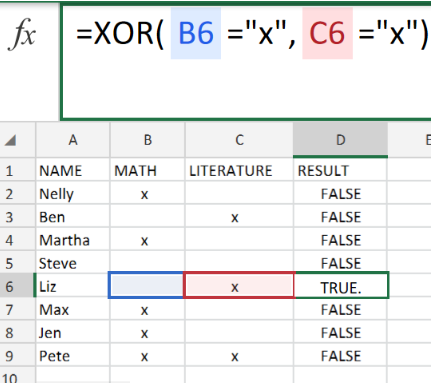Figure 1. One or The Other Not Both in Excel.

Let’s say we have two items and we want to SELECT EITHER OF THEM BUT NOT BOTH of two items, we will be using the Excel XOR function.

## Generic Formula

`=XOR(criteria1,criteria2)`

Just one criterion is allowed to be fulfilled.

## How to use the XOR Function in Excel.

We are going to utilize the XOR Function in 3 simple steps.

1. Collect the available dates and arrange within the labeled columns of our worksheet.

See example illustrated below;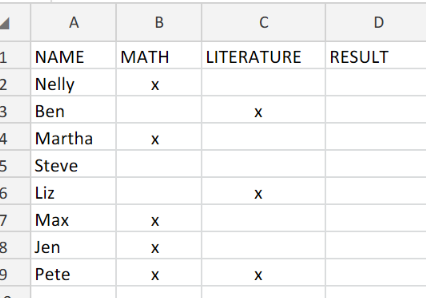Figure 2. One or The Other Not Both in Excel.

Our purpose here is for the XOR Function to return as TRUE when one of MATH and LITERATURE is marked “x” and return as FALSE if none of MATH or LITERATURE is marked “x” and also return as FALSE if both MATH and LITERATURE have an”x”.

1. In our example above, the XOR formula we will enter into cell D2 is as follows;

`=XOR(B2="x",C2="x")`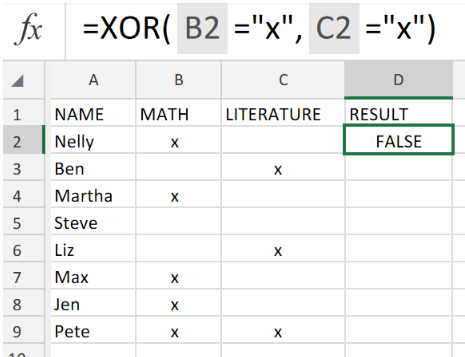Figure 3. One or The Other Not Both in Excel.

1. Edit the formula in cell D2 above, and enter into the other cells in the RESULT column to get the desired results.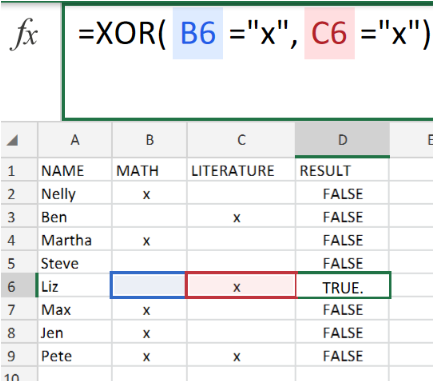Figure 4. One or The Other Not Both in Excel.

The XOR Function one criteria and then the next criteria and gives us a TRUE or FALSE outcome.

The behavior of the XOR Function is called an “exclusive” behavior; in which just one outcome can be TRUE. Never both.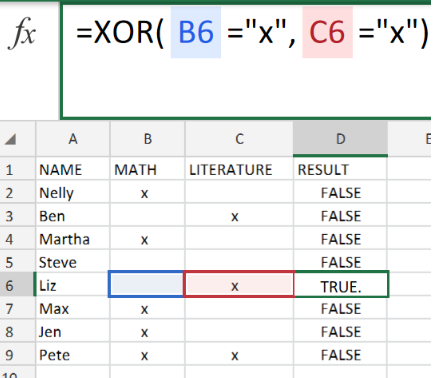Figure 5. Final Result.

## Instant Connection to an Expert through our Excelchat Service:

Our live Excelchat Service is here for you. We have Excel Experts available 24/7 to answer any Excel questions you may have. Guaranteed connection within 30 seconds and a customized solution for you within 20 minutes

### Did this post not answer your question? Get a solution from connecting with the expert.Another blog reader asked this question today on Excelchat:
Solution examplesUse the Vlookup Function to complete the "employee" column of table 2. Use "job Id" from table 2 as your lookup_value(s) and table 1 as your reference.
Solved by C. H. in 16 minsIf a cell in another sheet is populated I need a vlookup done. If the cell is not populated I need the cell to return blank.
Solved by T. D. in 60 minsI am trying to make a chart that turns a week range red if nothing is entered in the range. If something is entered then I would like it to turn green. Please Help
Solved by E. U. in 43 minsI need a check box to show/hide an answer of an if function
Solved by Z. U. in 23 minsHow do you get excel to ignore a formula until cell contains a value i.e.(if B1 is 1000, don't subtract C1 from B1 for a sum in C2 (resulting in -1000), until a value is first put in C1. ANOTHER EXAMPLE Don't add the sum of D5 and D6 in D7 if D5 and D6 are blank, preventing D7 (and E7 and G7...) from showing up as 0.00 ). I would like totals to remain blank and not the whole row showing 0.00 until a number is placed to complete the formula
Solved by V. J. in 28 mins## Subscribe to Excelchat.coAnother blog reader asked this question today on Excelchat: